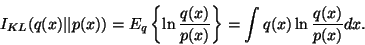Next: Cost function Up: BAYESIAN LEARNING IN PRACTICE Previous: Minimum message length inference

## Ensemble learning

Ensemble learning is a technique for parametric approximation of the posterior probability where fitting the parametric approximation to the actual posterior probability is achieved by minimising their misfit. The misfit is measured with Kullback-Leibler information , also known as relative or cross entropy. It is a measure suited for comparing probability distributions and, more importantly, it can be computed efficiently in practice if the approximation is chosen to be simple enough.

The Kullback-Leibler information between two probability density functions q(x) and p(x) is(14)

It has the following interpretation: suppose we are picking samples from distribution q(x), Kullback-Leibler information then measures the average amount of information the samples give for deciding that the samples are not from distribution p(x). If q(x) and p(x)are the same, then the amount of information is zero. On the other hand, if q(x) gives finite probability mass to samples for which p(x) gives zero probability, then a single such sample will reveal that the samples are not taken from p(x) and the average information is infinite.

Regarding the approximation of posterior probability, the most important benefit of ensemble learning is that Kullback-Leibler information is sensitive to probability mass and therefore the search for good models focuses on the models which have large probability mass as opposed to probability density. The drawback is that in order for ensemble learning to be computationally efficient, the approximation of the posterior needs to have a simple factorial structure. This means that most dependences between various parameters cannot be estimated. On the other hand, it should be possible to use ensemble learning instead of MAP estimation as the first stage in Laplace's method.

In the present form, the method was first presented by Hinton and van Camp  and the name ensemble learning was given by MacKay in . Ensemble learning can also be seen as a variational method  and it also has a connection to the EM algorithm .Next: Cost function Up: BAYESIAN LEARNING IN PRACTICE Previous: Minimum message length inference
Harri Valpola
2000-10-31